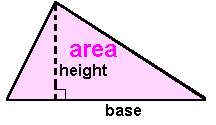## Functions and Operations

 A Short Table of Functions Midpoint of segment Diagonal of a Rectangle Distance between 2 points &Derivation of the Distance Formula Hypotenuse of a Right Triangle

 1. The absolute value of a number, |n|. Enter negatives as "-x" rather than "- x" ||

 2. The square root of a number,(n). The domain on this page is nonnegative values.3. The perimeter, P(L, W) = 2(L) + 2(W), or the
area, A(L, W) = (L)(W), of a rectangle.Width: Lenght:

is

4. Since all sides of a square are the same length,
the length equals the width, L = W.
The perimeter is four times the lenght:
P(L, W) = 2(L) + 2(W) = 2(L) + 2(L) = 4(L)
The area is the length squared:
A(L, W) = (L)(W) = (L)(L) = L²
The perimeter, P(L) = 4(L) or the
area, A(L) = L², of a square rectangle.Lenght:

is

5. Circles are describe in terms of their radius or diameter.
The circumference is the perimeter of a circle.The circumference,
in terms of radius,or
in terms of diameter,The area,
in terms of radius,or
in terms of diameter,Using a radius of:       The is square units.     The is units.
 Using a diameter of:       The is square units.     The is units.

5.1 Circle's arcs and sectors

Though radians make these formulas MUCH easier and clearer, this part of the page uses only degrees to measure angles and builds the conversion from degree to radian into the formulas. In the list of units shown below and the other images, the conversion formula is visible. The radians page also has a calculator to convert from radians (based on a circle's angle measure of 2) to degrees (based on a circle's angle measure of 360°).For the full story, see Arc Formulas and Rational, and Sector Formulas and Rational.

Click on the image below to see an enlargement of the formulas that are used here.Input the data. radius = central angle measure in degrees = time (if needed) = Press button to obtain the computed measure. arc length, s, units of length linear speed (unit of length)/(unit of time) angular speed degrees/(unit of time) area of sector square units

6.1 The midpoint of a segment,
M(x1,y1,x2,y2)= ( (x1+x2)/2, (y1+y2)/2 ).First Endpoint: (, ) Other Endpoint: (, )  Midpoint Coordinates: (, )

6.2 The diagonal of a rectangle,
D(L, W)=(L² + W²).Width: Lenght:

6.5. The are of a triangle, A(b, h)= (½)(b)(h).height: base:

6.75. The area of a trapezoid,
A(b1, b2, h)= (b1 + b2)(h)/2.height:   base:   other base:

7. Distance between 2 points:
Each point is named by one, two, or three coordinates.The distance formulas used for a line, a plane, or in space..
 From: () to () From: (, ) to (, ) From: (, , ) to (, , )
Click on the image to enlarge it.8. A Number to a Power. Please do not use fractions. Rewrite the fraction as a decimal or integer number. x:       y:
 9. The log of a number,The domain on this page is positive values. Rounding of the result is necessary.()

10. The volume of a rectangular prism, V(l,w, h)= lwh.
The volume of a rectangular pyramid, V(l,w, h)= lwh/3.lenght:   width:  height: cubic units   cubic units

11. The volume of a cylinder, V(r, h)=r²h.
The volume of a cone, V(r, h)=r²h/3.radius:   height: cubic units   cubic units© 2009, 2019 A. Azzolino www.mathnstuff.com/math/spoken/here/2class/80/math.htm